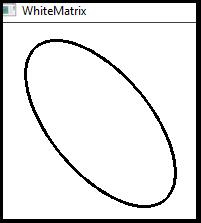# How to draw an Ellipse in OpenCV using C++?

To draw an ellipse, we need a center, major axis and minor axis. That means we need three parameters for the ellipse. We need a matrix where we will draw the ellipse, and we need to declare line thickness and line color.

When we want to draw an ellipse using OpenCV, we have to mention the angle of rotation, and there are two additional parameter starting point and ending point. To call 'ellipse()' function, we need to include<imgproc.hpp> header file.

The basic syntax of this method is as follows −

## Syntax

ellipse(whiteMatrix, center, xy,angle, starting_point, ending_point, line_Color,thickness);

The following program shows how to draw an ellipse in OpenCV.

## Example

#include<iostream>
#include<opencv2/highgui/highgui.hpp>
#include<opencv2/imgproc/imgproc.hpp>
using namespace cv;
using namespace std;
int main() {
Mat whiteMatrix(200, 200, CV_8UC3, Scalar(255, 255, 255));//Declaring a white matrix
Point center(100, 100);//Declaring the center point
Size xy(100, 50);//Declaring the major and minor axis of the ellipse//
int angle = 50;//angle of rotation//
int starting_point = 0;//Starting point of the ellipse//
int ending_point = 360;//Ending point of the ellipse//
Scalar line_Color(0, 0, 0);//Color of the Ellipse//
int thickness = 2;//thickens of the line//
namedWindow("whiteMatrix");//Declaring a window to show the ellipse//
ellipse(whiteMatrix, center, xy,angle, starting_point, ending_point,   line_Color,thickness);//Drawing the ellipse
imshow("WhiteMatrix", whiteMatrix);//Showing the ellipse
waitKey(0);//Waiting for Keystroke
return 0;
}

## Output